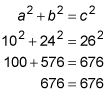##### Geometry: 1,001 Practice Problems For Dummies (+ Free Online Practice)You can classify equilateral, isosceles, scalene, and right triangles based on their side measurements. The following practice questions ask you to identify a triangle using algebra.

## Practice questions

Refer to the triangle PER. Use the following information to calculate the length of each side of the triangle and classify the triangle as isosceles, equilateral, scalene, and/or right.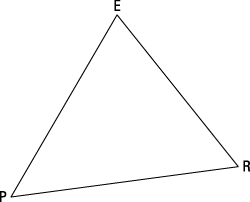1. The perimeter of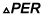is 108 units. The three sides of the triangle are represented by

PE = 5x + 11

ER = 7x + 1

RP = 8x – 4

Classify this triangle.

2. The perimeter ofis 60 units. The three sides of the triangle are represented by

PE = 2a + 8

ER = 2a – 6

RP = 3a + 2

Classify this triangle.

1. Equilateral

The given perimeter means that all three sides of the triangle add up to 108: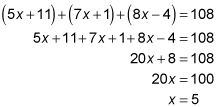Find the length of each side of the triangle by plugging in 5 for x: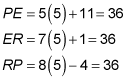Because all three sides of the triangle are equal, the triangle is equilateral.

2. Scalene right

The given perimeter means that all three sides of the triangle add up to 60: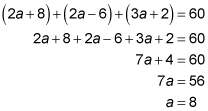Find the length of each side of the triangle by plugging in 8 for a: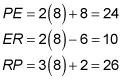The three sides of the triangle are all different in length, so the triangle is scalene.

Because the three sides of the triangle satisfy the Pythagorean theorem, the triangle is also a right triangle: